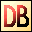DelphiBasics
 ArcSinFunction The Arc Sine of a number, returned in radians Math unit
 function ArcSin(const Number Extended):Extended;
Description
The ArcSin function is a mathematical function giving the value in radians of the Arc Sine of Number.

Notes
The System unit supports only the following angular functions:

ArcTan
Sin
Cos

Related commands
 ArcCos The Arc Cosine of a number, returned in radians ArcTan The Arc Tangent of a number, returned in radians Cos The Cosine of a number Sin The Sine of a number Tan The Tangent of a numberDownload this web site as a Windows program.

 Example code : The ArcSin of 0.5 gives 30 degrees var   float : single; begin   // The ArcSin of 0.5 should give 30 degrees   float := ArcSin(0.5);   float := RadToDeg(float);  // Convert result to degrees   ShowMessage('ArcSin of 0.5 = '+FloatToStr(float)+' degrees'); end; Show full unit code ArcSin of 0.5 = 30 degrees